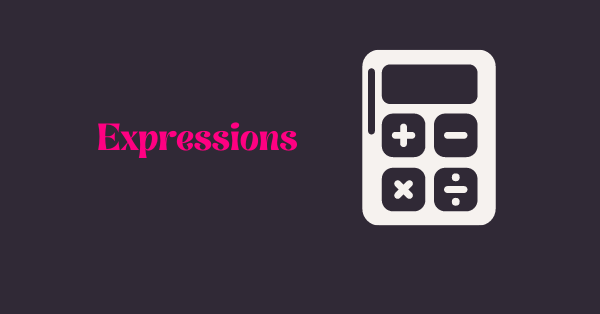# Using Expressions to create Scoring in surveysAug 9, 2022 · 3 mins readDo you want mathematical calculations to be performed while handling and creating surveys? Mathematical expressions will you get there easily. The good news is BlockSurvey supports mathematical expressions. Read on to learn more about that.

## What are expressions?

Expressions are mathematical statements. It is a combination of terms that are combined using mathematical operations like subtraction, addition, multiplication, and division. They are made up of constants and variables. Expressions are used to perform mathematical operations. In surveys, expressions are used to constantly evaluate a value that is related to the survey.

## What is scoring in surveys?

A scored survey is a questionnaire in which you assign points or scores to answer options. Scored surveys are used in online quizzes, tests, or any assessment. With scored surveys, you can learn more about the respondents' knowledge and their satisfaction level. They can also be used as screening tests. A typical use case of the scoring survey is an online quiz.

A scored survey does not have any right or wrong answers. Each answer is assigned a score that is fixed. Different points are assigned to each option. Generally, scores are not shown to the respondents at the end of the survey. A quiz is similar to a scored survey except for the individual scores of each question to create a final score. The final score is usually shared with the respondent.

## What is the difference between expressions and scoring?

In BlockSurvey, we have already created a variable called score. This score is the sum of all the questions in your quiz (that is a quiz-like survey). In addition to scores, one can create other variables and expressions. The newly created variables and expressions can be of use to solve other point problems with the survey.

## Using expression types

When you use mathematical expressions, you create unique mathematical calculations. BlockSurvey can evaluate expressions in a snap of the finger. I will walk you through how to use it.

Let us say we have 5 products. The cost of each product is 100. We calculate the total by multiplying no. of products and cost. In this case, the total is 5 * 100, which is 500.

Now we can use the Total as @Total in the Welcome screen, Thank you screen, or in questions. Similarly there is a default variable Score, which is the total of values assigned to all questions.

Blocksurvey allows many mathematical operations like

addition (+), subtraction (-), multiplication(*), division(/), Reminder(%)

Exponentiation(**), And(&), Or(|)

All expressions that are executable inside the javascript eval() function can be added in Blocksurvey. This operation can be addition, subtraction, multiplication, division, or any other.

## How to set up scoring using expressions in your surveys

In the builder, go to the Variables tab and hit expressions. As you can see below, BlockSurvey has a variable score to tally up the points assigned to each question.

Go to the Assignment tab of each question and assign points as below. We checking the answer to a question. In this case, we are checking if the capital of India is Delhi. If the answer is Delhi, we are assigning 1 point for the correct answer. Similarly go to each question, check the answers and assign points. Each question can have different points as well. In this case, we are assigning 1 point to all question.

In the Thank you screen, we can refer the score using @ symbol as below.

## How to create and use score groups

We can use score groups to score different sections using different variables.

In our example, the first 2 questions are about Indian GK, 3rd and 4th questions are about World GK. We want to score Indian GK and World GK separately. The World GK questions are stored in a separate variable called “WorldQuiz”.

In this way, we can add the points of questions 3 and 4 in a separate variable “WorldQuiz”. This is how we can form score groups. In the response we can see the results.

In the “World GK section”, question 4 is answered wrongly twice. So the score is 1 each time for that section.

## How to view the scores from your survey responses

The scores can be viewed through the survey responses. You can find the answer to each question and the total score assigned to each taking of the quiz.

Now that you know how to use score groups and set up scoring in your surveys, you can start creating a quiz and scoring on your own.

### Interested in other BlockSurvey features? Visit BlockSurvey features template gallery.

Get future posts from BlockSurvey

### Like what you see? Share with a friend.### Sarath Shyamson

Sarath Shyamson is the customer facing person in the BlockSurvey ecosystem and also heads the outreach team. He enjoys volunteering for the church choir and loves spending time with his two year old son.